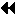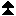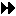Wachowicz's Web Workout WEB EXERCISES FOR DISCERNING FINANCE STUDENTS

### PART II: VALUATION Chapter 3: The Time Value of Money Visit Wachowicz's Web World

 Exercise 3.1 Compound Interest: Future Value of Single Amount

Business Finance Online features a number of Time Value of Money (TVM) calculators.

The boxed Q&A in the "Compound Interest" section of textbook Chapter 3 solves a time value of money problem (i.e., the future value of \$58 at 5 percent compound annual interest in 215 years {211 years, if you are using the 11th edition of the book}) by making use of a Future Value Interest Factor Table. Use the Time Value of Money Calculator to more accurately solve this problem. (Note: You can use this calculator when the present value (PV) is known and you are solving for the future value (FV).)

Q. What answer do you get using the PV calculator and how does this compare with the answer found in the text? (The dollar difference -- caused by rounding error -- between the two answers should be quite small.)

 Exercise 3.2 Compound Interest: Present Value of Single Amount

The Time Value of Money Calculator can be used when the future value (FV) is known and you are solving for the present value (PV).

Q. Using this calculator, what is the present value of \$2,000 to be received at the end of 10 years, discounted at an 8 percent compound annual interest rate?

 Exercise 3.3 Compound Interest: Annuities

The Time Value of Money Calculator can be used when the periodic payment/receipt value is known and you are solving for either the future or present value of an ordinary annuity.

In Figures 3-4 and 3-5 in the text, you will find the solutions to two ordinary annuity problems (i.e., the future/present value of \$1,000 a year for 3 years at 8 percent compound annual interest). The textbook solutions employ Future/Present Value Interest Factor of an Annuity Tables. Use the Time Value of Money Calculator to more accurately solve these same two problems.

Q. What answers do you get using the Payment Value Calculator? How do these answers compare to the answers found in the textbook? (The dollar differences -- caused by rounding error -- between the textbook answers and the answers provided by the payment value calculator should be quite small.)

 Exercise 3.4 Compounding More Than Once a Year

The Time Value of Money Calculator can be used when the present value (PV) is known and you are solving for the future value (FV. This calculator can also handle calculations where compounding occurs more frequently than once a year.

Q. Suppose that interest is paid quarterly. What is the future value of \$100 at the end of 3 years where the nominal, or stated, annual interest rate is 8 percent? Compare your answer with that found in the "Compounding More Than Once a Year" section in the text's Chapter 3. Are the answers similar?

 Exercise 3.5 Amortizing a Loan

The Amortization Calculator calculates your periodic payment for a given loan balance and stated, or nominal, interest rate. In addition, it creates a loan amortization schedule.
http://ray.met.fsu.edu/~bret/amortize.html

Q. Establish a loan amortization schedule for each of the following two loans. Now, what is the monthly payment for each loan?

 a. A 36-month loan of \$8,000 with equal installment payments at the end of each month. The stated, or nominal, compound annual interest rate is 12 percent. b. A 25-year mortgage loan of \$184,000 at a 10 percent compound annual interest rate with equal installment payments at the end of each year.Previous Page |Back to Main Index | Next Page